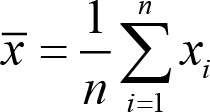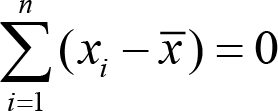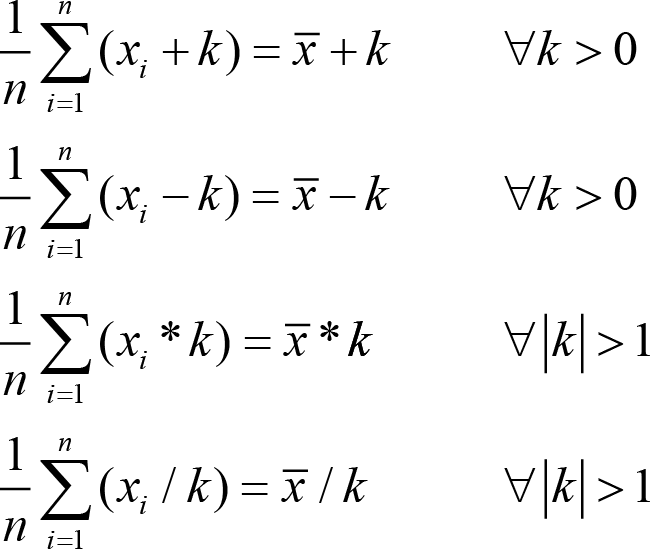# Mean Properties

Mean Properties

By Russel Winder

Property based testing is all the rage. Russel Winder walks us through an example of properties an arithmetic mean function should have.

In the article Testing Propositions [ Winder16 ], I used the example of factorial to introduce proposition-based testing. One of the criticisms from an unnamed reviewer was that it was not clear what constituted a proposition; the test code looked very much like the implementation code, confusing the message. The reviewer had clearly missed the point, which most likely must indicate a problem with the presentation and/or the example chosen in the article. This short article is to try and present an example to address that reviewer’s valid, and important, point.

## A really (really) small problem

Let us consider the calculation of the mean of a set of data. Mathematically we would write:where x ̄ represents the mean of the dataset comprising all the values x i . The mathematical statement leads us inexorably to an algorithm for computing the mean of a given data set: using Python 1 we implement a function ``` mean ``` as shown in Listing 1. Of course many people might have just written the code as shown in Listing 2 and whilst correct, this code is likely to be much slower than using NumPy. 2

 ```# python_numpy.py import numpy mean = numpy.mean ``` Listing 1
 ```# python_pure.py def mean(data): return sum(data) / len(data) ``` Listing 2

The question now is obviously: how can we test these implementations?

## Do we have to?

The insightful reader will already have spotted that there is probably not a testing obligation for us with the ``` mean ``` function as implemented in Listing 1. The code uses assignment (which should work because the Python compiler and virtual machine 3 implementers have tested that it works correctly) and a reference to ``` numpy.mean ``` which should work because the NumPy implementers should have tested that that function works correctly.

But what about Listing 2? Is there a testing obligation given that the ``` sum ``` function, the ``` len ``` function and the ``` / ``` (division) operator are all Python features and the Python language people should have tests for all of them? Is this function not correct simply by observation, and that it reflects so easily the mathematical definition? No. We must test this function. 4

## Yes, we will test things

So what tests can we do in the property-based rather than example-based philosophy? Well we have two implementations of the same functionality so maybe we can test that they both produce the same answer. The property being tested here is that all correct implementations give the same answer. Using [ Hypothesis ] and [ PyTest ], I came up with the test code shown in Listing 3.

 ```from math import isclose from hypothesis import given from hypothesis.strategies import lists, floats import python_numpy import python_pure lower_bound = -1e5 upper_bound = 1e5 effectively_zero = 1e-3 @given(lists( floats(min_value=lower_bound, max_value=upper_bound). filter(lambda x: abs(x) > effectively_zero))) def test_the_two_implementations_give_the_same_result(data): assert isclose(python_numpy.mean(data), python_pure.mean(data)) ``` Listing 3

There is clearly a lot going on in this code. There is a single test function, but we use the Hypothesis ``` given ``` decorator and ``` lists ``` and ``` floats ``` strategies to automatically generate (more or less randomly) lists of floating point values that the test is run on. Most of the work is in conditioning the ``` float ``` values that we allow in the test: the float values are constrained to the ranges [-1e5, -1e-3] and [1e-3, 1e5], via the combination of ``` min_value ``` and ``` max_value ``` constraints in the ``` floats ``` strategy combined with the ``` filter ``` strategy that applies a predicate to remove values in the range [-1e-3, 1e-3]. Values close to zero are not allowed since hardware floating point values close to zero generally cause serious problems with any and all expression evaluation and hence testing. 1 Similarly, admitting very large floating point values allows Hypothesis to easily discover values that hardware floating point expression evaluation cannot cope with – again resulting in problems of testing nothing to do with the actual properties under test. 2 So, for the purposes of testing, we stick with floating point values of about eight significant digits to try and ensure we do not get rounding errors in the hardware evaluation that falsify our properties due to the behaviour of floating point hardware rather than a failing of the property.

The test itself eschews asserting equality of two hardware floating point values, as this is clearly not a thing any right thinking programmer would ever dream would work. 3 Instead the ``` math.isclose ``` predicate is used to determine if the values are close enough to each other to be deemed equal.

Anyone ‘on the ball’ will already have realised that there was going to be an error executing this test, even with the carefully constructed test. When pytest is run on the test code we get:

```  dataset = []
def mean(dataset):
>   return sum(dataset) / len(dataset)
E   ZeroDivisionError: division by zero```

One up to Hypothesis for finding that the empty dataset breaks our implementation.

If we took a ‘Just fix the tests so they are green’ approach 4 we might just change the tests to that as seen in Listing 4. The empty dataset case is separated out and the ``` @given ``` decorator is extended to required that only lists with at least one item are generated.

 ```from math import isclose from numpy import isnan from hypothesis import given from hypothesis.strategies import lists, floats from pytest import raises import python_numpy import python_pure lower_bound = -1e5 upper_bound = 1e5 effectively_zero = 1e-3 def test_numpy_mean_return_nan_on_no_data(): assert isnan(python_numpy.mean([])) def test_our_mean_raises_exception_on_no_data(): with raises(ZeroDivisionError): python_pure.mean([]) @given(lists( floats(min_value=lower_bound, max_value=upper_bound). filter(lambda x: abs(x) > effectively_zero), min_size=1)) def test_the_two_implementations_give_the_same_result(data): assert isclose(python_numpy.mean(data), python_pure.mean(data)) ``` Listing 4

## But is this the right behaviour?

The question we have to ask is whether this behaviour of the implementation of Listing 2 with empty lists is the right behaviour. Indeed this should have been the question asked instead of just patching the test in the first place. 5

We note that ``` numpy.mean ``` returns ``` NaN ``` for empty data rather than throwing an exception. In the original submission of this article, I amended the ``` pure_python.mean ``` to return ``` NaN ``` , just to be consistent with ``` python_numpy.mean ``` . The reviewers, though, were fairly unanimous that NumPy was doing the wrong thing, that mean of an empty dataset should be undefined, i.e. raise an exception. This viewpoint is encouraged when looking at the ``` statistics ``` module in the Python standard library (from Python 3.4 onwards). Its ``` mean ``` function definitely raises an exception on no data. So, let’s treat the ``` numpy.mean ``` behaviour as an aberration: let’s change the implementation of our pure Python ``` mean ``` function to that shown in Listing 5 and make the appropriate change to the test for empty data, as shown in Listing 6.

 ```# python_pure_corrected.py def mean(data): if len(data) == 0: raise ValueError('Cannot take mean of no data.') return sum(data) / len(data) ``` Listing 5
 ```from math import isclose from numpy import isnan from hypothesis import given from hypothesis.strategies import lists, floats from pytest import raises import python_numpy import python_pure_corrected lower_bound = -1e5 upper_bound = 1e5 effectively_zero = 1e-3 def test_numpy_mean_return_nan_on_no_data(): assert isnan(python_numpy.mean([])) def test_our_mean_raises_exception_on_no_data(): with raises(ValueError) as error: python_pure_corrected.mean([]) assert error.value == 'Cannot take mean of no data.' @given(lists( floats(min_value=lower_bound, max_value=upper_bound). filter(lambda x: abs(x) > effectively_zero), min_size=1)) def test_the_two_implementations_give_the_same_result(data): assert isclose(python_numpy.mean(data), python_pure_corrected.mean(data)) ``` Listing 6

## But what are the properties?

None of this has though really opened up the question of properties of the computation: we have only example-based tests for the empty dataset, and a comparison test to test the equivalence of different implementations. So what are the properties of the mean calculation that we can test in the form of property-based tests for each implementation separately?

A search of Google 6 should always turn up the property that:i.e. the sum of the differences of individual items from the mean should be zero. Given a dataset and a putative mean calculation function, we can test that this property is not falsifiable. Further delving into the notion of ‘properties of the mean’ may well also turn up the following properties:So here we have a number of expressions (that we can treat as predicates, i.e. properties) that can be evaluated, that our putative mean implementation should pass. 7 All of them are exactly the sort of property that Hypothesis can test using its random generation of values from a set.

## And the result is…

Putting all this together I present the test as shown in Listing 7. 8> , 9 , 10

 ```from math import fabs, isclose import operator import statistics from numpy import isnan from hypothesis import given, settings from hypothesis.strategies import lists, floats from pytest import mark, raises import python_numpy import python_pure_corrected implementations = (python_numpy.mean, python_pure_corrected.mean, statistics.mean) operators = (operator.add, operator.sub, operator.mul, lambda a, b: a / b) lower_bound = -1e5 upper_bound = 1e5 effectively_zero = 1e-3 def test_numpy_mean_return_nan_on_no_data(): assert isnan(python_numpy.mean([])) def test_our_mean_raises_exception_on_no_data(): with raises(ValueError) as error: python_pure_corrected.mean([]) assert error.value == 'Cannot take mean of no data.' def test_statistics_mean_raises_exception_on_no_data(): with raises(statistics.StatisticsError): statistics.mean([]) @mark.parametrize('implementation', implementations) @given(lists( floats(min_value=lower_bound, max_value=upper_bound) .filter(lambda x: fabs(x) > effectively_zero), min_size=1, max_size=100)) def test_sum_of_data_minus_mean_is_zero(implementation, data): x_bar = implementation(data) assert sum(x - x_bar for x in data) < effectively_zero @mark.parametrize('implementation', implementations) @mark.parametrize('op', operators) @given(lists( floats(min_value=lower_bound, max_value=upper_bound). filter(lambda x: fabs(x) > effectively_zero). map(lambda x: round(x, 3)), min_size=1), floats(min_value=2.0, max_value=upper_bound), ) def test_mean_of_changed_data_obeys_property(implementation, op, data, offset): x_bar = implementation(data) x_bar_offset = implementation([op(x, offset) for x in data]) assert isclose(x_bar_offset, op(x_bar, offset)) ``` Listing 7

We have the example-driven test of the empty list case, as previously.

The test of equivalence of different implementations is replaced by tests of the properties for each implementation separately: ``` test_sum_of_data_minus_mean_is_zero ``` realises the first equation, and ``` test_mean_of_offset_data_is_correct ``` handles all four ‘offset testing’ equations. Both of these functions are parameterised using the ``` pytest.mark.parametrize ``` decorator so as to create tests for each of the ``` mean ``` implementations being tested, three in this case. The ``` test_mean_offset_data_is_correct ``` test function is also parameterised over the arithmetic operations +, -, *, and /, 11 implementing the four ‘offset testing’ equations. The selection of lists of floating point values is very much as previously, but with the creation of the offset value added as a second parameter to the ``` given ``` decorator.

All the tests pass. 12

So, hopefully, this short article has ‘filled in the gaps’ left by the previous article, about what a property is and what property-based testing is about.

## Final thought

Is the mean of a dataset the only function that has the properties tested here?

## References

[Hypothesis] http://hypothesis.works/

[PyTest] http://pytest.org/

1. Allow values close to zero in the sample set and Hypothesis will always find a case that falsifies any property.
2. This property of hardware floating point numbers and Hypothesis (or any random test value generator with a good search algorithm) is well understood and is unavoidable, hence having to take great care conditioning the floating point values selected.
3. Any programmer claiming competence that uses equality between floating point values clearly requires re-education on this point.
4. Anyone taking this approach in real life is definitely doing it wrong, even though we all know this is what actually happens all too often. Clearly we should fight against this approach at all times.
5. Except then I wouldn’t have had a chance to chide people taking the ‘just fix the tests so they are green’ approach.
6. Yes, Google searching can actually turn up useful facts, as well as satire, spoof, and fictional stuff. And pictures of cats, obviously.
7. In the early draft of this article, I failed to note the constraints on k in the equations, although I had implemented the constraints in the code. This raised a debate amongst the reviewers that there were only two, not four, equations. Both sides were right because the presentation was, at that time, inconsistent. The question was which consistency to go with. For a small number of minor ‘hidden agenda’ points, I have gone with four equations, but going with two equations and not restricting the domain would be an equally valid way forward.
8. I have added ``` statistics.mean ``` in here as well, just for good measure. Formally, fairly useless, or it should be, but fun nonetheless.
9. Actually, we really should note that testing ``` numpy.mean ``` and ``` statistics.mean ``` here is completely superfluous and totally redundant in terms of testing obligation. It is done here to show that all the implementations do, in fact, pass the tests.
10. Thanks to D R MacIver, the author of Hypothesis, for being around on Twitter on 2017-01-01 to fix a serious architectural failing with the draft of this code. In summary: each test should have one and only one given decorator.
11. Python provides functional forms of the +, -, and * operators, but not /, hence the lambda function for division.
12. At the time of submission of this article, running on Debian Sid with Python 3.5.2, PyTest 3.0.5, and Hypothesis 3.6.0, anyway.